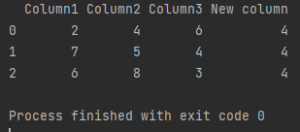Pandas How To Uncategorized How to add a column

# How to add a column

Here’s the tutorial on how to add a column to the existing dataframe in Python Pandas library.

You probably already know how to create a dataframe and want to start working with it.

Dataframe in Pandas is a very flexible form of data presentation. It is easy to filter, aggregate and group data. You can also remove and add rows and columns.

To extend your dataframe and insert an additional column, proceed as shown below.

Here is my dataframe. We will fill it with sample data. You can of course use your own dataframe.

```import pandas as pd

my_df = pd.DataFrame({'Column1': ['2', '7', '6'],
'Column2': ['4', '5', '8'],
'Column3': ['6', '4', '3']})

print(my_df)
```

To add a column, define it first.

```new_column = ['4', '4', '4']
```

You can also add it based on an already existing data structure, e.g. a list that you have already defined. I am presenting here the simplest possible example to make it easier for you to understand.

## How to add a column

To add a previously defined column assign the variable in which you defined it to your dataframe and indicate that you want it to be your new column as below.

```my_df["New column"] = new_column
```

The code from my example looks like this:

```import pandas as pd

my_df = pd.DataFrame({'Column1': ['2', '7', '6'],
'Column2': ['4', '5', '8'],
'Column3': ['6', '4', '3']})

new_column = ['4', '4', '4']

my_df["New column"] = new_column

print(my_df)

```The new column is appended to the right side of the dataframe by default. You can use the insert method to add a column at a specific position in the dataframe.

Now you know how to add a column to a dataframe in Pandas. Browse through my site to solve any further issues you encounter with Pandas.

Tags:

## How to read multiple Excel sheets in PandasHow to read multiple Excel sheets in Pandas

Xlsx files are very often the source of data that you analyze in Pandas. Today you will learn how to read multiple Excel sheets in Pandas.

## Which data structure of the Pandas work with 3D data?Which data structure of the Pandas work with 3D data?

Pandas is a Python library that provides tools for data analysis and manipulation. Its core data structure is the DataFrame, which is a 2D table-like structure that can hold data